Courses

# Concentration Of Solution Notes | EduRev

## Class 12 : Concentration Of Solution Notes | EduRev

The document Concentration Of Solution Notes | EduRev is a part of the Class 12 Course Chemistry for JEE.
All you need of Class 12 at this link: Class 12

CONCENTRATION OF SOLUTION
We can calculate the concentration of solutions by various methods. Let’s study each method and determine the formulas for this method.

• Percent by weight
• Percent by volume
• Percent by weight/volume
• Molarity
• Molality
• Normality
• Mole Fraction
• ppm
• Formality
• Strength (g/L)

1. Mass/Weight Percentage or Percentage by Mass/Weight
It is the amount of solute in grams present in 100 grams of the solution. Therefore, the formula will be:
Mass Percentage = Mass of Solute/Mass of Solution × 100
= mass of Solute/Mass of Solute + Mass of Solvent × 100
= Mass of Solute/Volume of Solution + Density of Solution × 100
The ratio mass of solute to the mass of the solvent is the mass fraction. Thus, the mass percentage of solute = Mass fraction × 100. 10% solution of sugar by mass means that 10 grams of sugar is present in 100 grams of the solution, i.e., we have dissolved 10 grams of sugar in 90 grams of water.

2. Volume Percentage
It is the volume of solute in mL present in 100 mL solution. The formula will be:
Volume Percentage = Volume of solute/Volume of Solution × 100
10% solution of HCl by volume means that 10 mL of liquid HCl is present in 100 mL of the solution.

3. Mass by Volume Percentage
It is the mass of solute present in 100 mL of solution. We can calculate the mass of the solute using the volume percentage.
The formula would be:
Mass by Volume Percentage = Mass of Solute/Volume of Solution × 100
It changes on changing temperature.
Example: A 10% (w/v) urea solution. = 10 gm of urea is present in 100 mL of solution.
Density of solution is required to calculate weight of solute and weight of solvent.

4. Molarity
The molarity of a solution gives the number of gram molecules of the solute present in one litre of the solution.
Molarity(M) = Number of moles of solute/Volume of Solution in L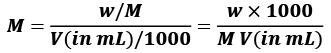w = Mass of solute in grams
M = molecular weight of solute in gm/mol.
V = volume of solution in ml.
Example. 1 mol L-1 solution of KCl means that 1 mol of KCl is dissolved in 1 L of water.
Unit of molarity: mol L-1

• Molarity is most common way of representing the concentration of solution.
• Molarity is depend on temperature as M ∝ 1/T
• When a solution is diluted (x times), its molarity also decreases (by x times)

Example. Calculate the molarity of a solution containing 5 g of NaOH in 450 mL solution.
Solution.
Moles of NaOH = 5g/40 g mol-1 = 0.125 mol
Volume of the solution in litres = 450 mL / 1000 mL L-1
Using equation (2.8),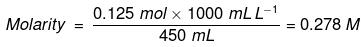= 0.278 mol L–1
= 0.278 mol dm–3

5. Molality
Molality of a solution is the number of moles of solute dissolved in 1 Kg of the solvent.
Molality (m) = Number of moles of solute/Mass of Solvent in Kg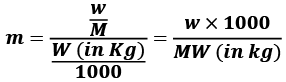w = mass of solute in grams
M = molecular wt of solute
W = mass of solvent in gram.
Thus, if one gram molecule of a solute is present in 1 kg of the solvent, the concentration of solutions is said to be one molal.
The unit of molarity is mol kg-1.

• Molality is the most convenient method to express the concentration of solutions because it involves the mass of liquids rather than their volumes. It is also independent of the variation in temperature.

Example. Calculate molality of 2.5 g of ethanoic acid (CH3COOH) in 75 g of benzene.
Solution.
Molar mass of C2H4O2 : 12 × 2 + 1 × 4 + 16 × 2 = 60 g mol–1
Moles of C2H4O2 = 2.5/60 g mol-1 = 0.0417 mol
Mass of benzene in kg = 75 g/1000 g kg–1 = 75 × 10–3 kg
Molality of C2H4O2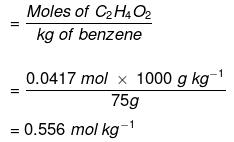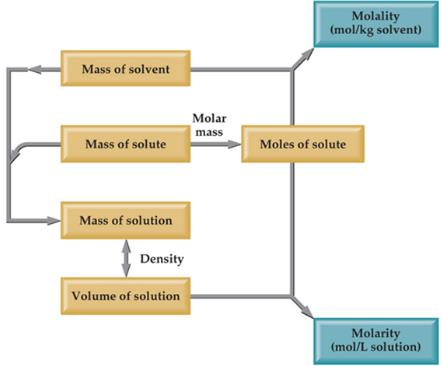6. Normality
The normality of a solution gives the number of gram equivalents of the solute present in one litre of the solution.
Normality (N) = Number of gram equivalent of solute/Volume of Solution in L
Equivalent mass = Molar mass/n - factor

No. of equivalent = Mass of solute/equivalent mass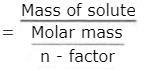= No. of moles of solute x n - factorw = mass of solute in gram
V = volume of solution in ml
E = equivalent wt of solute
Thus, if one gram equivalent of a solute is present in one litre of the solution,  the concentration of solutions is said to be 1 normal.

• 1N = Normal = One gram equivalent of the solute per litre of solution = Normality is 1
• N/2 = Seminormal = 0.5 g equivalent of the solute per litre of solution = Normality is 0.5
• N/10 = Decinormal = 0.1 g equivalent of the solute per litre of solution = Normality is 0.1
• N/100 = Centinormal = 0.01 g equivalent of the solute per litre of solution = Normality is 0.01
• N/1000 = Millinormal = 0.001 g equivalent of the solute per litre of solution = Normality is 0.001

Relationship between normality and other Concentration Terms

• Molarity × Molecular mass= Strength of the solution (g/L)
• Normality × Equivalent mass = Strength of the solution (g/L)
• Molarity × Molecular mass= Normality x Equivalent mass
• Normality = n × Molarity

7. Mole Fraction
Mole fraction can be defined as the ratio of number of moles of the component in the solution to the total number of moles of all components in the solution.

• It is denoted by the alphabet x and subscript written on the right hand side of x denotes the component of which mole fraction is being calculated.
• Mathematically, Mole fraction of a component =
Number of moles of the component/Total number of moles of all components
• Let us consider a binary mixture of A and B. let the number of moles of A and B be nA and nB respectively, then
Mole fraction of A = xA = nA/ nA + nB
Mole fraction of B = xB = nA/ nA + nB
• For solution where number of components = i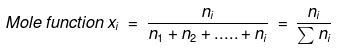• In a given solution sum of the mole fractions of all the components is

unity.
Mathematically, x1 + x2 + x3 …. + xi =1

Example. Calculate the mole fraction of ethylene glycol (C2H6O2) in a solution containing 20% of C2H6O2 by mass.

Solution.
Assume that we have 100 g of solution (one can start with any amount of solution because the results obtained will be the same). Solution will contain 20 g of ethylene glycol and 80 g of water.
Molar mass of C2H6O= 12 × 2 + 1 × 6 + 16 × 2 = 62 g mol–1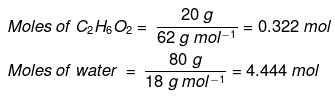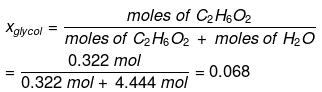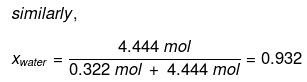Mole fraction of water can also be calculated as: 1 – 0.068 = 0.932.

8. Parts per million (ppm)
When a solute is present in trace quantities, it is convenient to express the concentration of solutions in parts per million (ppm). The formula is as follows: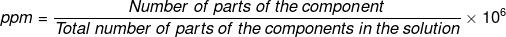In case of mass, we may express it as: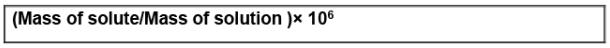In case of volume, we may express it as: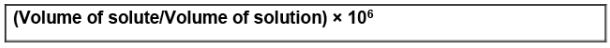So, we can express the concentration of solutions in parts per million as mass to mass, volume to volume and mass to volume form.

• Atmospheric pollution in cities is also expressed in ppm by volume.
• It refers to the volume of the pollutant in 106 units of volume.
• 10 ppm of SO2 in the air means 10 mL of SO2 is present in 106 mL of air.

9. Formality

• It is the number of mass in grams present per litre of solution.
• In case, formula mass is equal to molecular mass, formality is equal to molarity.
• Like molarity and normality, the formality is also dependent on temperature. It is used for ionic compounds in which there is no existence of a molecule.
• A mole of ionic compounds is called formal and molarity as the formality.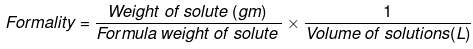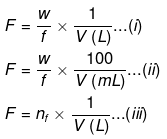Where,

• w = weight of solute,
• f = formula weight of solute
• V= volume of solution
• nf = no. of gram formula weight

10. Strength (g/L)
Weight of solute per litre (1000 mL) of solution.
Example: 10% w/v sucrose solution, then specify its concentration in g/L
In 100 mL solution, weight of sucrose = 10 g
Therefore, in 1000mL solution= 10/100 = 100 g
Hence strength will be 100g/L

MOLARITY OF MIXING
Let there be three samples of solution (containing same solvent and solute) with their molarities M1 ,M2 ,M3 and volumes V1 ,V2 ,V3 respectively.
These solutions are mixed; molarity of mixed solution may be given as: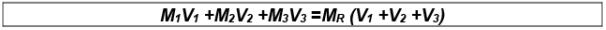where, MR is the Resultant molarity
V+ V2 + V3 = Resultant volume after mixing
Note: Molarity is dependent on volume; therefore, it depends on temperature.

 1M molar solution, i.e, molarity is l 0.5 M or M/2 Semimolar 0.1 M or M/2 Decimolar 0.01 M or M/100 Centimolar 0.001 M or M/100 Millimolar

DILUTION FORMULA

M1V1 = M2V2

(Concentrated solution)  (Diluted solution)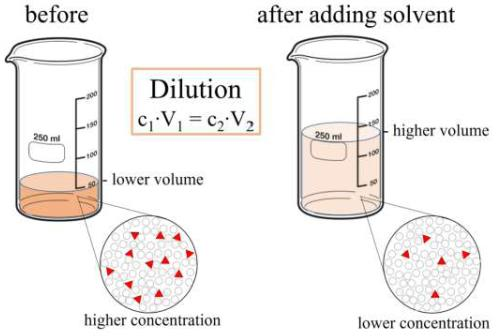Problem 1. Calculate the masses of cane sugar and water required to prepare 250 g of 25% cane sugar solution.
Solution.
Mass percentage of cane sugar = 25
We know that,
Mass percentage = Mass of solute/Mass of solution × 100
25 = Mass of cane sugar/250g × 100
Mass of cane sugar = 25 ×250/100 = 62.5 g
Mass of water (250 -62.5)g = 187.5 g

Problem 2. A tank is charged with a mixture of 1.0 x 103 mol of oxygen and 4.5 x 103 mol of helium. Calculate the mole fraction of each gas in the mixture.
Solution.
The given parameters are
nHe = 4.5 x 103 mol and nO2 = 1.0 x 103 mol
Mole fraction can be calculated as
xHe = 4.5 x 103 mol / (4.5 x 103 mol + 1.0 x 103 mol)
=4.5 mol / 5.5 mol = 0.82
xO2 = 2.0 x 103 mol / (4.5 x 103 mol + 1.0 x 103 mol)
= 1.0 x 103 / 5.5 x 103
= 0.18

Problem 3. The density of a solution containing 13% by mass of sulphuric acid is 1.09 g/mL. Calculate the molarity and normality of the solution.
Solution.
Volume of 100 g of the solution
= 100/d = 100/1.09 mL
= 100/1.09 × 1000 litre
= 1/1.09 × 10 litre
Number of moles of H2so4 in 100 g of the solution = 13/98
Molarity = No. of moles of H2So4/Volume of Solu. in litre = 13/98 × 1.09 × 10/1
= 1.445 M
We know that,
Normality = Molarity × n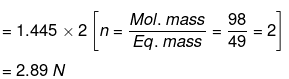Problem 4. What is the mole fraction of carbon tetrachloride (CCl4) in solution if 3.47 moles of CCl4 is dissolved in 8.54 moles of benzene (C6H6)?
Solution.
Mole Fraction CCI4 (XCCI4) = moles of CCI4/total moles
XCCI4 = 3.47 moles CCI4/3.47 moles CCI4 + 8.54 moles C6H6
XCCI4 = 0.289

Problem 5. What is the mole fraction of formaldehyde (CH2O) in solution if 25.7 grams of CH2O is dissolved in 3.25 moles of carbon tetrachloride (CCl4)?
Solution.
The grams of acetone will need to be converted into moles in order to solve for mole fraction.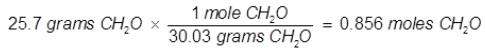Mole Fraction CH2O(XCH2O) = moles of CH2O/total moles
XCH2O = 0.856 moles CH2O/0.856 moles CH2O + 3.25 moles CCI4
XCH2O = 0.208

Problem 6. 0.100 mole of NaCl is dissolved into 100.0 grams of pure H2O. What is the mole fraction of NaCl?
Solution.
100.0 g / 18.0 g mol-1 = 5.56 mol of H2O
Add that to the 0.100 mol of NaCl = 5.56 + 0.100 = 5.66
mol total Mole fraction of NaCl = 0.100 mol / 5.66 mol = 0.018
The mole fraction of the H2O:
5.56 mol / 5.66 mol = 0.982

Problem 7. A solution is prepared by mixing 25.0 g of water, H2O, and 25.0 g of ethanol, C2H5OH. Determine the mole fractions of each substance.
Solution.
(1) Determine the moles of each substance:
H2O ⇒ 25.0 g / 18.0 g/mol = 1.34 mol
C2H5OH ⇒ 25.0 g / 46.07 g/mol = 0.543 mol
(2) Determine mole fractions:
H2O ⇒ 1.34 mol / (1.34 mol + 0.543 mol) = 0.71
C2H5OH ⇒ 0.543 mol / (1.34 mol + 0.543 mol) = 0.29

Problem 8. A solution contains 10.0 g pentane, 10.0 g hexane and 10.0 g benzene. What is the mole fraction of hexane?
Solution.
Try it yourself Using hints given below !
(1) You need to determine the moles of pentane, hexane and benzene: to do this, you need the molecular weights. Here are the formulas:
pentane: C5H12
hexane: C6H14
benzene: C6H6
(2) When you have the moles of each, add them together.
(3) Then, divide the moles of hexane by the total.

Problem 9. The molality of an aqueous solution of sugar (C12H22O11) is 1.62m. Calculate the mole fractions of sugar and water.
Solution.
(1) Molality is moles solute / kg of solvent. Therefore we know our solution is:
1.62 mol C12H22O11
1.00 kg = 1000 g of water
(2) Calculate the moles of water present:
1000 g / 18.0152 g/mol = 55.50868 mol
(3) Determine the mole fraction of the sugar:
1.62 mol / (1.62 mol + 55.50868 mol) = 0.028357 = 0.0284 (to three sf)
(4) You can calculate the mole fraction of the water by subtraction.

Problem 10. How many grams of water must be used to dissolve 100.0 grams of sucrose (C12H22O11) to prepare a 0.020 mole fraction of sucrose in the solution?
Solution.
(1) Determine moles of sucrose:
100.0 g / 342.2948 g/mol = 0.292145835 mol
(2) Determine moles of water required to make the solution 0.020 mole fraction of sucrose:
0.020 = 0.292 / (0.292 + x)
(0.020) (0.292 + x) = 0.292
0.00584 + 0.02x = 0.292
0.02x = 0.28616
x = 14.308 mol of H2O
Comment: you can also do this:
0.292 is to 0.02 as x is to 0.98
(3) Determine grams of water:
14.308 mol x 18.015 g/mol = 258.0 g

Problem 11. Surprisingly, water (in the form of ice) is slightly soluble in liquid nitrogen. At -196 °C, (the boiling point of liquid nitrogen) the mole fraction of water in a saturated solution is 1.00 x 10-5. Compute the mass of water that can dissolve in 1.00 kg of boiling liquid nitrogen.
Solution.
(1) Use the definition of mole fraction to set up the following:
χwater = moles of water / (moles of water + moles of nitrogen)
1.00 x 10-5 = x / (x + 71.3944041)
I'm going to carry some guard digits until the end of the calculation.
(2) Some algebra: (1.00 x 10-5) (x) + 7.139440411 x 10-4 = x
0.99999x = 7.139440411 x 10-4
x = 7.139511806 x 10-4 mol of H2O
(3) Calculate grams of water from moles of water:
7.139511806 x 10-4 mol x 18.0152 g/mol = 1.2862 x 10-2 g
1.29 x 10-2 g (to three sf)

Problem 12. What is the mole fraction of cinnamic acid in a mixture that is 50.0% weight urea in cinnamic acid (urea = 60.06 g/mol; cinnamic acid = 148.16 g/mol)
Solution.
(1) Let us assume 100.0 g of this mixture are present. Therefore:
50.0 g is urea
50.0 g is cinnamic
(2) Convert grams to moles: urea:
50.0 g / 60.06 g/mol = 0.8325 mol
cinnamic acid: 50.0 g / 148.16 g/mol = 0.3375 mol
(3) Determine mole fraction of cinnamic acid:
0.3375 mol / 1.1700 mol = 0.2885

Problem 13. 5 g of NaCl is dissolved in 1000 g of water. If the density of the resulting solution is 0.997 g per cc, calculate molality, molarity, normality and mole fraction of the solute.
Solution.
Mole of NaCl = 5/58.5 = 0.0854 (Mol. wt. of NaCl = 58.5)
Molality = Moles/Wt. of solvent in gram x 1000
= 0.0854/1000 x 1000 = 0.0854 m
Volume of the solution= Wt. of solution in gram/Density in gram / cc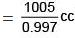Again by definition
Molarity = Moles/Volume of solution in litre
= 0.0854/1.008
= 0.085 M
∴ Normality = 0.085 N (for NaCl, eq. wt. = mol. wt)
Further, Mole of H2O = 1000/18 = 55.55
(1000 gram of water = 1000 ml of water, because density = 1 g/cc)
Total mole = Mole of NaCl + Mole of H2O
= .0854 + 55.55 = 55.6354
Mole fraction of NaCl = 7.85/98.66 = 0.0854/55.6409 = 1.535 x 10–3

Problem 14. If 20 ml of ethanol (density  = 0.7893 gm / ml) is mixed with 40 ml of water (density = 0.9971 gm/ml) at 25°C, the final solution has density of 0.9571 gm / ml. Calculate the percentage change in total volume of mixing. Also calculate the molality of alcohol in the final solution.
Solution.
Mass of ethanol = v x d
W = 20 × 0.7893 gm = 15.786 gm
Mass of water = v × d
= 40 x 0.9971 gm = 39.884 gm
Total volume = 60 ml
Total mass = 15.786 + 39.884 = 55.67 gm
Let the volume of solution = x ml
X = mass/density = 55.67/0.9571 = 58.165 ml
Change in volume = 60 – 58.165 = 1.835 ml
% change in volume = 1.835/60 x 100
= 3.05%
Molality, m = w/m x 1000/W (in gm) = 15.786/46 x 1000/39.884

Problem 15. Calculate the mole fraction of benzene in solution containing 30% by mass in carbon tetrachloride.
Solution.
Total mass of the solution = 100 g
Mass of benzene = 30 g.
∴ Mass of carbon tetrachloride = (100 - 30)g = 70 g
Molar mass of benzene (C6H6) = (6 × 12 + 6 × 1) g mol– 1 = 78 g mol-1
∴ Number of moles of C6H6 =30/78 mol = 0.3846 mol
Molar mass of carbon tetrachloride (CCl4) = 1 × 12 + 4 × 355 = 154 g mol-1
∴ Number of moles of CCl4 = 70/154 mol = 0.4545 mol
Mole fraction of C6H6 =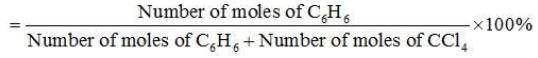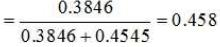Problem 16. Determine the mole fraction of methanol CH3OH and water in a solution prepared by dissolving 4.5 g of alcohol in 40 g of H2O. Molar mass of H2O is 18 and Molar mass of CH3OH is 32.
Solution.
Moles of CH3OH = 4.5/32 = 0.14
mole Moles of H2O = 40/18 = 2.2 moles
Therefore, according to the equation
mole fraction of CH3OH = 0.14/2.2 + 0.14
= 0.061

Offer running on EduRev: Apply code STAYHOME200 to get INR 200 off on our premium plan EduRev Infinity!

## Chemistry for JEE

223 videos|452 docs|334 tests

,

,

,

,

,

,

,

,

,

,

,

,

,

,

,

,

,

,

,

,

,

;